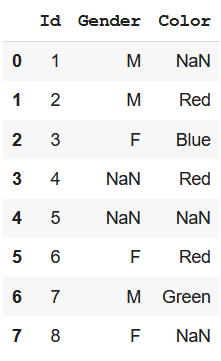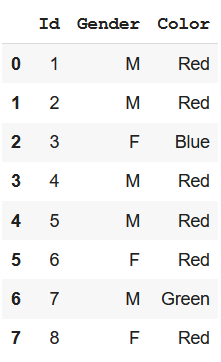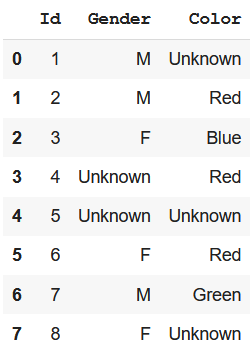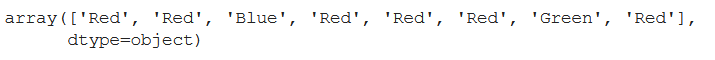GeeksforGeeks App
Open AppBrowser
Continue

# Pandas – Filling NaN in Categorical data

Real-world data is full of missing values. In order to work on them, we need to impute these missing values and draw meaningful conclusions from them. In this article, we will discuss how to fill NaN values in Categorical Data. In the case of categorical features, we cannot use statistical imputation methods.

Let’s first create a sample dataset to understand methods of filling missing values:

## Python3

 `# import modules``import` `numpy as np``import` `pandas as pd` `# create dataset``data ``=` `{``'Id'``: [``1``, ``2``, ``3``, ``4``, ``5``, ``6``, ``7``, ``8``],``        ` `        ``'Gender'``: [``'M'``, ``'M'``, ``'F'``, np.nan,``                   ``np.nan, ``'F'``, ``'M'``, ``'F'``],``        ` `        ``'Color'``: [np.nan, ``"Red"``, ``"Blue"``,``                  ``"Red"``, np.nan, ``"Red"``,``                  ``"Green"``, np.nan]}` `# convert to data frame``df ``=` `pd.DataFrame(data)``display(df)`

Output:To fill missing values in Categorical features, we can follow either of the approaches mentioned below –

Method 1: Filling with most occurring class

One approach to fill these missing values can be to replace them with the most common or occurring class. We can do this by taking the index of the most common class which can be determined by using value_counts() method. Let’s see the example of how it works:

## Python3

 `# filling with most common class``df_clean ``=` `df.``apply``(``lambda` `x: x.fillna(x.value_counts().index[``0``]))``df_clean`

Output:Method 2: Filling with unknown class

At times, the missing information is valuable itself, and to impute it with the most common class won’t be appropriate. In such a case, we can replace them with a value like “Unknown” or “Missing” using the fillna() method. Let’s look at an example of this –

## Python3

 `# filling with Unknown class``df_clean ``=` `df.fillna(``"Unknown"``)``df_clean`

Output:Method 3: Using Categorical Imputer of sklearn-pandas library

We have scikit learn imputer, but it works only for numerical data. So we have sklearn_pandas with the transformer equivalent to that, which can work with string data. It replaces missing values with the most frequent ones in that column. Let’s see an example of replacing NaN values of “Color” column –

## Python3

 `# using sklearn-pandas package``from` `sklearn_pandas ``import` `CategoricalImputer` `# handling NaN values``imputer ``=` `CategoricalImputer()``data ``=` `np.array(df[``'Color'``], dtype``=``object``)``imputer.fit_transform(data)`

Output:My Personal Notes arrow_drop_up